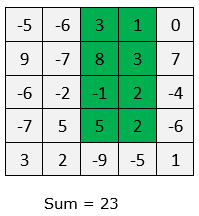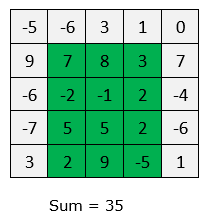# Find Maximum Sum Submatrix present in a given matrix

Given an M x M matrix, find maximum sum submatrix present in it.

For example, maximum sum submatrix is highlighted in green in below matrices –The idea is to pre-process the matrix. We take an auxiliary matrix S[][] where S[i][j] will store the sum of the elements in matrix from (0, 0) to (i-1, j-1). The idea is similar to this post –

After we have pre-processed the matrix to create the sum matrix, we consider every sub-matrix formed by row i to j and column m to n calculate sub-matrix sum in constant time using below relation –

sub-matrix sum = S[j+1][n+1] – S[j+1][m] – S[i][n+1] + S[i][m];

If the sub-matrix sum is more than maximum found so far, we update the maximum sum. We can also store the sub-matrix coordinates to print the maximum sum submatrix.

## Java

Output:

Output:

Sub-matrix is formed by row 1 to 4 and column from 1 to 3
The maximum sum of sub-matrix is 35

The time complexity of above solution is O(n4) and auxiliary space used by the program is O(n2). We can solve this problem in O(n3) time using Kadane’s algorithm. We will soon publish this solution in separate post.(1 votes, average: 5.00 out of 5)Loading...

Please use our online compiler to post code in comments. To contribute, get in touch with us.
Like us? Please spread the word and help us grow. Happy coding 🙂